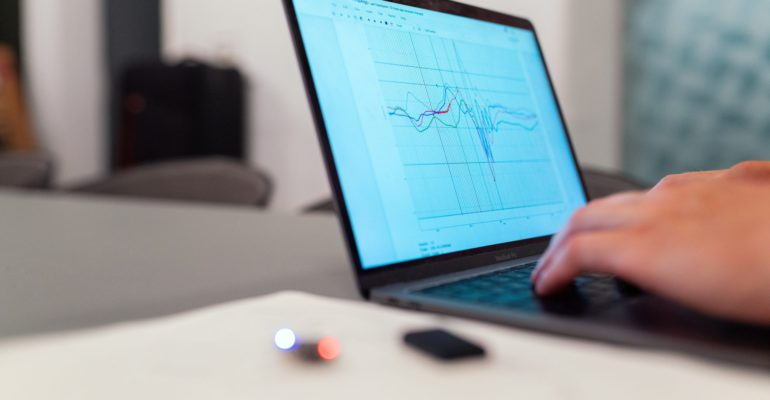Thanks to theidioms.com

### Infosys Tech Scholars Scholarship## Working with images in Matplotlib# Working with images in Matplotlib

Greetings! Some links on this site are affiliate links. That means that, if you choose to make a purchase, The Click Reader may earn a small commission at no extra cost to you. We greatly appreciate your support!

Matplotlib provides an easy interface to work with images. In this chapter, we will get familiar with reading, plotting and manipulating images in matplotlib.

#### How to read images in Matplotlib?

To work with images we first need to import the image data into Numpy arrays using the imread() function of Matplotlib Image (imported below as mpimg).

```# Libraries/Modules import conventions
import matplotlib.image as mpimg

# Read a network/local image and store as numpy array

# Printing the image type
print(type(img))```
`Output: <class 'numpy.ndarray'>`

#### How to plot images in Matplotlib?

Images can be plotted in Matplotlib with the help of the imshow() function of Matplotlib pyplot as shown in the following example:

```# Libraries/Modules import conventions
import matplotlib.image as mpimg

# Read a network/local image and store as numpy array

# Show the image stored in the numpy array
imgplot = plt.imshow(img)```

Since the image is basically stored as pixel values in a NumPy array, we can change the values of the pixels to manipulate the image. The following example changes the color of the image:

```# Libraries/Modules import conventions
import matplotlib.image as mpimg

# Read a network/local image and store as numpy array

# Apply pseudocolor schemes to image plots
manipulated_img = img[:, :, 0] # array slicing operation

# Show the image stored in the manipulated numpy array
imgplot = plt.imshow(manipulated_img)```

Now we know how to read, display and manipulate an image in Matplotlib. For more details on working with images, this Matplotlib guide can be useful.

Also, congratulations on completing this course on Matplotlib!

#### End of Course

With this, we have come to the end of our Matplotlib for Data Science Course. We hope that this course helped you as a stepping stone towards your Data Science journey with Python. If you have any questions or feedback, please feel free to let us know in the comment section.

Also, now that you are able to code using Matplotlib, you can enroll in a domain-specific course by going through all of our intermediate and expert courses.

(As a reminder, we are constantly updating our courses so make sure to check in on a future date to find more resources introduced in this course.)Do you want to learn Python, Data Science, and Machine Learning while getting certified? Here are some best selling Datacamp courses that we recommend you enroll in:

1. Introduction to Python (Free Course) - 1,000,000+ students already enrolled!
2. Introduction to Data Science  in Python- 400,000+ students already enrolled!
3. Introduction to TensorFlow for Deep Learning with Python - 90,000+ students already enrolled!
4. Data Science and Machine Learning Bootcamp with R - 70,000+ students already enrolled!